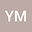•There are different numerical models, such as the transmission-line matrix model or partially uniform knee model used to predict Schumann radiation. This report introduces a new idea, and reasoning to the previously stated idea of locating Schumann resonances on a single particle’s radiation pattern using a Golden ratio and their Octave, triad relationship. In addition, this different prediction method for Schumann resonances derived from the first principle fundamental physics combining both particle radiation patterns and the mathematical concept of the golden ratio spiral that expands at the rate of the golden ratio. The idea of golden ratio spiral allows locating Schumann resonant frequencies on particle’s radiation patterns. The Octaves allows us to predict the magnitude of other Schumann resonances on the radiation pattern of a single accelerated charged particle conveniently by knowing the value of the initial Schumann resonant frequency. In addition, it also allows us to find and match Schumann resonances that are on the same radiation lobe. Furthermore, it is important to find Schumann octaves as they propagate in the same direction and have a higher likelihood of wave interference. Method of Triads together with Octaves helps to predict magnitude and direction of Schumann resonant points without needing to refer to a radiation pattern plot. As the golden ratio seems to be part of the Schumann resonances, it is helpful in understanding to know why this is the case. The main method used in the reasoning of the existence of golden ratio in Schumann resonances is the eigenfrequency modes, $\sqrt{n(n+1)}$ in the spherical harmonic model. It has been found that eigenfrequency modes have two a start off points, $n_0 = 0$ or $n_0 = \frac{\sqrt{5}-1}{2}$ where the non-zero one is exactly the golden ratio. This allows to extend the existing eigenfrequency modes to $\sqrt{(n_0+n)^2+(n_o+n)}$ in order to explain why golden ratio exist within Schumann resonances.